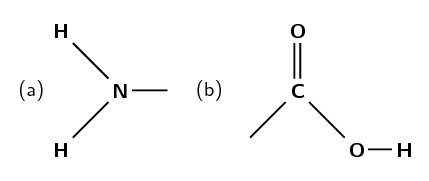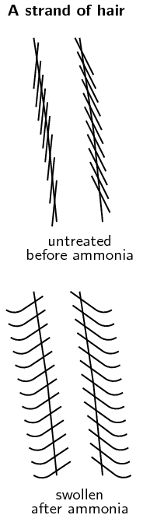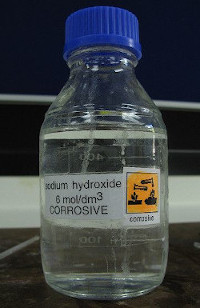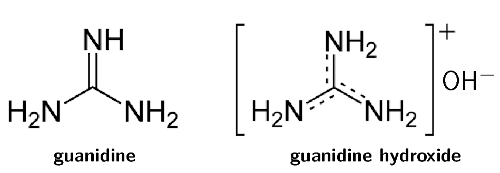We think you are located in United States. Is this correct?

# Test yourself now

High marks in science are the key to your success and future plans. Test yourself and learn more on Siyavula Practice.

## 9.5 Applications of acids and bases (ESCPN)

### The production of chlorine (ESCPP)

The chlorine-alkali (chloralkali) industry is an important part of the chemical industry for the production of chlorine and sodium hydroxide. The most common method involves the electrolysis of a concentrated aqueous solution of sodium chloride ($$\text{NaCl}$$), which is known as brine. For more information on electrolysis see Chapter 13.

The chemical reactions that take place in this process are:

$$2\text{Cl}^{-}(\text{aq})$$ $$\to$$ $$\text{Cl}_{2}(\text{g}) + 2\text{e}^{-}$$

$$2\text{H}_{2}\text{O}(\text{ℓ}) + 2\text{e}^{-}$$ $$\to$$ $$\text{H}_{2}(\text{g}) + 2\text{OH}^{-}(\text{aq})$$

There are also $$\text{Na}^{+}$$ ions from the $$\text{NaCl}$$ in the solution. If the products are kept separate (to prevent a reaction between the chlorine and hydroxide) the $$\text{Na}^{+}$$ will react with the hydroxide ions making the overall reaction as follows:

$$2\text{NaCl}(\text{aq}) + 2\text{H}_{2}\text{O}(\text{ℓ})$$ $$\to$$ $$\text{Cl}_{2}(\text{g}) + \text{H}_{2}(\text{g}) + 2\text{NaOH}(\text{aq})$$

The uses of chlorine include:

• the purification of water

• as a disinfectant

• in the production of:

• hypochlorous acid (used to kill bacteria in drinking water), chloroform, carbon tetrachloride

• paper, food

• antiseptics, insecticides, medicines, textiles

• paints, petroleum products, solvents, plastics (such as polyvinyl chloride)

The uses of sodium hydroxide include:

• making soap and other cleaning agents

• purification of bauxite (the ore of aluminium)

• making paper

• making rayon (artificial silk)

If the chlorine and hydroxide ions are not kept separate (i.e. are allowed to react), and the temperature is kept below $$\text{60}$$ $$\text{℃}$$, then the following occurs:

$$\text{Cl}_{2}(\text{g}) + 2\text{OH}^{-}(\text{aq})$$ $$\to$$ $$\text{Cl}^{-}(\text{aq}) + \text{ClO}^{-}(\text{aq}) + \text{H}_{2}\text{O}(\text{ℓ})$$

The balanced chemical equation with the inclusion of the $$\text{Na}^{+}$$ ions is:

$$\text{Cl}_{2}(\text{g}) + 2\text{NaOH}(\text{aq})$$ $$\to$$ $$\text{NaCl}(\text{aq}) + \text{NaClO}(\text{aq}) + \text{H}_{2}\text{O}(\text{ℓ})$$

The uses of $$\text{NaClO}$$ (sodium hypochlorite) include:

• use in bleaches, disinfectants, and water treatments

• use during root canal surgery and to neutralise nerve agents

If the temperature is above $$\text{60}$$ $$\text{℃}$$ and mixing occurs, then:

$$3\text{Cl}_{2}(\text{g}) + 6\text{OH}^{-}(\text{aq})$$ $$\to$$ $$5\text{Cl}^{-}(\text{aq}) + \text{ClO}_{3}(\text{aq}) + 3\text{H}_{2}\text{O}(\text{ℓ})$$

And the balanced chemical equation including $$\text{Na}^{+}$$ ions is:

$$3\text{Cl}_{2}(\text{g}) + 6\text{NaOH}(\text{aq})$$ $$\to$$ $$5\text{NaCl}(\text{aq}) + \text{NaClO}_{3}(\text{aq}) + 3\text{H}_{2}\text{O}(\text{ℓ})$$

The uses of $$\text{NaClO}_{3}$$ (sodium chlorate) include:

• making chlorine dioxide

• use as a herbicide

• generating oxygen in chemical oxygen generators

If calcium chloride was used instead of sodium chloride, then the products would be calcium chloride, calcium hypochlorite and calcium chlorate. Similarly, if potassium chloride was used, the products would be potassium chloride, potassium hypochlorite and potassium chlorate.

### The chemistry of hair and hair products (ESCPQ)

Hair is primarily made up of a protein called keratin. Keratin is a macromolecule and consists largely of the amino acid cystine (an organic acid, see Figure 9.4).

Amino acids are composed of an amine group (Figure 9.5 (a)) and a carboxylic acid group (Figure 9.5 (b)) connected by a carbon chain.Figure 9.5: a) An amine functional group and b) a carboxylic acid functional group. Both of these are found in amino acids.

Proteins give hair a natural acidic pH of between $$\text{4}$$ and $$\text{5}$$. The following hair products alter this pH and do controlled damage to get the desired effect.

#### Permanent waving application

Another name for a permanent waving application is a perm. Ammonium thioglycolate is also known as a perm salt. The reversible reaction of ammonium thioglycolate (reactant) to form thioglycolic acid and free ammonia (product) is given below:

$$\text{HSCH}_{2}\text{COO}^{-}(\text{aq}) + \text{NH}_{4}^{+}(\text{aq})$$ $$\rightleftharpoons$$ $$\text{HSCH}_{2}\text{COOH}(\text{aq}) + \text{NH}_{3}(\text{aq})$$

There are four common steps in the perming process:

1. The basic ammonia swells the hair and makes it permeable.2. The thioglycolic acid reduces the sulfur-sulfur bonds in the cystine molecules in your hair. This reduces cystine to cysteine (Figure 9.6) and allows the hair structure to be changed.

This reaction occurs faster at higher temperatures, and the hair is generally heated at this time (e.g., on a hot curling iron).

3. The ammonium thioglycolate solution is washed out of the hair after the desired bond breaking has occurred.

4. The hair is treated with hydrogen peroxide ($$\text{H}_{2}\text{O}_{2}$$), which acts as a neutraliser. It oxidises the cysteine back to cystine (re-forming the sulfur-sulfur bonds). These bonds will form in the new positions formed during the ammonium thioglycolate application. These bonds then fix the hair in this new structure.

It is important to use the neutraliser as well as wash the hair thoroughly after a perm application. Excess exposure to the ammonium thioglycolate can cause scalp irritation, as well as permanent hair damage.

#### Hair relaxers

Hair relaxers work by doing controlled damage to your hair in much the same way as perms do. They reduce the cystine to cysteine and allow the hair structure to be changed (Figure 9.6).

There are two main types of hair relaxers: lye and no-lye. The lye hair relaxers contain sodium hydroxide ($$\text{NaOH}$$) and have a very high pH (in the range $$\text{12}$$ - $$\text{14}$$). These types of hair relaxers break bonds and strip the hair of its natural oils. Often heating is also used and this damages the hair even more.

$$\text{NaOH}$$ is used in drain cleaning products to dissolve hair, as well as in procedures that remove body hair.Remember that the pH scale goes from $$\text{0}$$ - $$\text{14}$$.

Due to increasing worry about the damage caused by lye hair relaxers, companies have produced no-lye hair relaxers. These still contain basic compounds and are still caustic, but their pH is not as high (pH in the range $$\text{9}$$ - $$\text{11}$$).

These products either use calcium hydroxide and guanidine carbonate (to form guanidine hydroxide, Figure 9.7) or lithium hydroxide. These are bases and act in a similar manner to sodium hydroxide. Although they are milder on hair than sodium hydroxide, they are still damaging. Another type of no-lye hair relaxer contains ammonium thioglycolate (the perm salt).Figure 9.7: Guanidine (left) and guanidine hydroxide (right). Guanidine hydroxide is formed from guanidine carbonate and calcium hydroxide.

Some comparisons between lye and no-lye hair relaxers are given in Table 9.10.

 Lye No-lye pH $$\text{12}$$ - $$\text{14}$$ $$\text{9}$$ - $$\text{11}$$ Benefits quick application time more gentle on scalp Problems high pH, very damaging to scalp and hair often left on too long, dries hair due to calcium build up

Table 9.10: Lye versus no-lye hair relaxers.

#### Colouring hair

There are many different types of hair colourants, ranging from permanent hair dye to temporary hair dye. Each of them works in a slightly different way.

• Permanent

In permanent hair dyes there are three main ingredients: a diamino compound (that means two of the amine groups shown in Figure 9.5 (a)); a coupling agent; and an oxidising agent.

The oxidising agent is generally hydrogen peroxide ($$\text{H}_{2}\text{O}_{2}$$). It oxidises the diamino compound to a state where it can bond with the coupling agent. This process also lightens the hair so that the dye will work more effectively. This new compound is then oxidised again to form the final dye.

Permanent dyes are basic with a pH between $$\text{9}$$ and$$\text{11}$$ (usually ammonia, $$\text{NH}_{3}$$). The purpose of the base is to open up the hair and allow the small dye molecules to penetrate.

• Demi-permanent

These dyes use ethanolamine or sodium carbonate as a base. These are less harsh bases than ammonia (pH between $$\text{8}$$ and $$\text{9}$$), and so do not allow the dye to penetrate as far into the hair.

They still contain a hydrogen peroxide developer that lightens the hair slightly. However, they cannot colour dark hair to a lighter colour. The hair colour will look more natural though, as the natural colour of the hair will affect the final dye. Demi-permanent dyes last a long time (at least $$\text{12}$$ washes), but will eventually wash out.

Demi-permanent hair dyes do not damage the hair as much as permanent dyes.

• Semi-permanent

These dyes contain molecules that are small enough to partially penetrate the hair without the need of a base to open the hair. They last for about $$\text{4}$$ - $$\text{5}$$ washes and have, at most, very low levels of hydrogen peroxide or ammonia. They will not lighten hair and the final colour depends a lot on the original colour and the porosity of the hair.

• Temporary

These are generally bright dyes. They are composed of large dye molecules, which are unable to penetrate the hair. These large molecules stick to the hair and usually can be removed with just one wash. If the hair is already dry or damaged the colour may last longer, as the damaged hair is more open and allows the dye molecules to penetrate.

It is worth noting that some of the possible side-effects of using perming applications, hair relaxers and hair colourants are:

• scalp irritation, skin burns, and permanent scarring

• deep ulcerations, dermatitis, skin drying and cracking

• irreversible baldness, eye damage or blindness

• weak, broken, or damaged hair

Although they are common house hold items, drain cleaners and caustic soda are extremely corrosive. Learners are working with a strong base and concentrated, strong bases can cause serious burns. Please remind the learners to be careful and wear the appropriate safety equipment when handling all chemicals, especially concentrated bases. The safety equipment includes gloves, safety glasses, and protective clothing.

## The effect of drain cleaner on hair

### Aim

To determine the effect of caustic soda or drain cleaner on hair.

### Apparatus

• A pile of hair from your hairbrush

• A drain cleaning product or caustic soda flakes

• A watch glass

Be careful when handling any drain cleaning products. They are very basic and can burn your skin. We suggest using gloves and safety glasses as well as protective clothing. Handle all chemicals with care.

### Method

1. Collect the hair from your hair brush for a week.

2. Place the hair on a watch glass.

3. If you have a liquid drain cleaning product perform step a. If you have caustic soda perform step b.

1. Carefully pour a drain cleaning product onto the pile of hair and observe.

2. Take the solid caustic soda and put a few flakes onto the hair. Boil some water, pour it carefully over the caustic soda and observe.

### Questions

• What is the main ingredient in the drain cleaning product and the caustic soda?

• What happened to the hair when this product was applied to it?

• What is the main ingredient of lye hair relaxers?

### Conclusions

You should have observed that the hair disintegrated when the drain cleaner was applied. The main ingredient in these cleaners is also the main ingredient in many hair relaxers, although the concentration is lower in the hair relaxers.